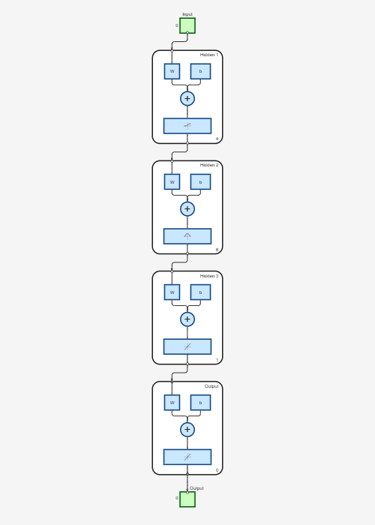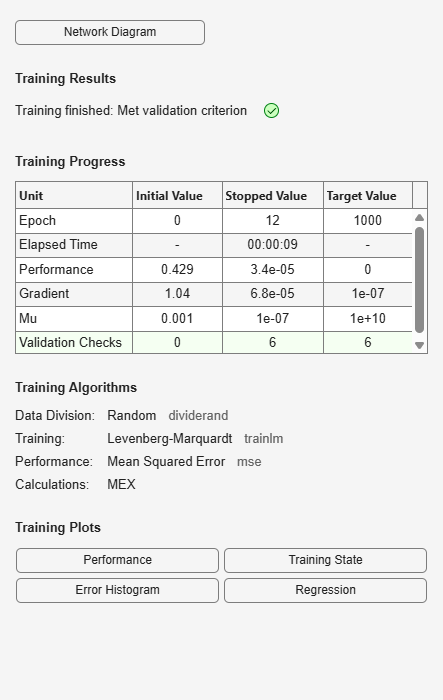idFeedforwardNetwork

Multilayer feedforward neural network mapping function for nonlinear ARX models (requires Deep Learning Toolbox)

Description

An idFeedforwardNetwork object implements a neural network function, and is a nonlinear mapping object for estimating nonlinear ARX models. This mapping object lets you use network (Deep Learning Toolbox) objects that are created using Deep Learning Toolbox™ in nonlinear ARX models.Mathematically, idFeedforwardNetwork is a function that maps m inputs X(t) = [x(t1),x2(t),…,xm(t)]T to a scalar output y(t), using a multilayer feedforward (static) neural network, as defined in Deep Learning Toolbox.

You create multi-layer feedforward neural networks by using commands such as feedforwardnet (Deep Learning Toolbox), cascadeforwardnet (Deep Learning Toolbox) and linearlayer (Deep Learning Toolbox). When you create the network,

• Designate the input and output sizes to be unknown by leaving them at the default value of zero (recommended method). When estimating a nonlinear ARX model using the nlarx command, the software automatically determines the input and output sizes of the network.

• Initialize the sizes manually by setting input and output ranges to m-by-2 and 1-by-2 matrices, respectively, where m is the number of nonlinear ARX model regressors and the range values are minimum and maximum values of regressors and output data, respectively.

Use evaluate(net_estimator,x) to compute the value of the function defined by the idFeedforwardNetwork object net_estimator at input value x. When used for nonlinear ARX model estimation, x represents the model regressors for the output for which the idFeedforwardNetwork object is assigned as the nonlinearity estimator.

You cannot use idFeedforwardNetwork when the Focus option in nlarxOptions is 'simulation' because the underlying network object is considered to be nondifferentiable for estimation. Minimization of simulation error requires differentiable nonlinear functions.

Use idFeedforwardNetwork as the value of the OutputFcn property of an idnlarx model. For example, specify idFeedforwardNetwork when you estimate an idnlarx model with the following command.

sys = nlarx(data,regressors,idFeedforwardNetwork)
When nlarx estimates the model, it essentially estimates the parameters of the idFeedforwardNetwork function.

Creation

Description

example

net_estimator = idFeedforwardNetwork(Network) creates a feedforward neural network mapping object that is based on the feedforward (static) network object Network that has been created using one of the neural network commands feedforwardnet, cascadeforwardnet, or linearlayer. Network must represent a static mapping between the inputs and output without I/O delays or feedback. The number of outputs of the network, if assigned, must be set to one. For a multiple-output nonlinear ARX models, create a separate idFeedforwardNetwork object for each output—that is, each element of the output function must represent a single-output network object.

Properties

expand all

Feedforward neural network object, typically created using feedforwardnet (Deep Learning Toolbox), cascadeforwardnet (Deep Learning Toolbox) or linearlayer (Deep Learning Toolbox).

Input signal information for signals used for estimation, specified as vectors of m property-specific values, where m is the number of input signals. The Input properties for each input signal are as follows:

• Name — Names of the input signals, specified as a 1-by-m string or character array, where m is the number of inputs

• Mean — Mean of the input signals, specified as a numeric scalar

• Range — Ranges of the input signals, specified as a 2-by-m numeric array that contains the minimum and maximum values

Option to train the neural network, specified as true or false. Set Free to false when the neural network you are using has already been trained and is known to provide good fit results. The Free property is especially useful when your idnlarx model has multiple outputs that each use a neural network. Setting Free to false for well trained networks allows processing time to be focused on the networks that do need training.

Output signal information, specified as property-specific values. The Output properties are as follows:

• Name — Name of the output signal, specified as a string or a character array

• Mean — Mean of the output signal, specified as a numeric scalar

• Range — Range of the output signal, specified as a 2-by-1 numeric array that contains the minimum and maximum values

Examples

collapse all

Create a neural network mapping object that uses a feedforward neural network with three hidden layers, transfer functions of types logsig, radbas,and purelin, and unknown input and output sizes.

Create a neural network.

net = feedforwardnet([4 6 1]);
net.layers{1}.transferFcn = 'logsig';
net.layers{3}.transferFcn = 'purelin';

View the network diagram.

view(net)Create a neural network mapping object.

net_estimator = idFeedforwardNetwork(net);

Create a single-layer, cascade-forward network with unknown input and output sizes and use this network for nonlinear ARX model estimation.

Create a cascade-forward neural network with 20 neurons and unknown input/output sizes.

Create a feedforward neural network mapping object.

net_estimator = idFeedforwardNetwork(net);

data = iddata(y,u,0.2);

Estimate a nonlinear ARX model sys.

sys = nlarx(data,[2 2 1],net_estimator);

Compare the model response to the measured output signal.

compare(data,sys)The plot shows good agreement between the measured signal and the simulated model output signal.

Initialize the input-output sizes of a two-layer feed-forward neural network based on estimation data, and use this network for nonlinear ARX estimation.

z7 = z7(1:200);

Create a template nonlinear ARX model with no nonlinearity.

model = idnlarx([4 4 4 1 1],[]);

This model has six regressors and is used to define the regressors. The range of regressor values for input-output data in z7 is then used to set the input ranges in the neural network object, as shown in the next steps.

Obtain the model regressor values.

R = getreg(model,'all',z7);
R = R.Variables;

Create a two-layer, feed-forward neural network and initialize the network input and output dimensions to 2 and 1, respectively. Use 5 neurons for first layer and 7 for second layer.

net = feedforwardnet([5 7]);

Determine input range.

InputRange = [min(R);max(R)].';

Initialize input dimensions of estimator.

net.inputs{1}.range = InputRange;

Determine output range.

OutputRange = [min(z7.OutputData),max(z7.OutputData)];

Initialize output dimensions of estimator and the choice of training function.

net.outputs{net.outputConnect}.range = OutputRange;
net.trainFcn = 'trainbfg';

Create a neural network nonlinearity estimator.

net_estimator = idFeedforwardNetwork(net);

Specify the nonlinearity estimator in the model.

model.Nonlinearity = net_estimator;

Estimate the parameters of the network to minimize the prediction error between data and model. Estimate model.

model = nlarx(z7,model);

Compare model's predicted response to measured output signal.

compare(z7(1:100),model,1)Algorithms

The nlarx command uses the train method of the network object, defined in the Deep Learning Toolbox software, to compute the network parameter values.

Compatibility Considerations

expand all

Not recommended starting in R2021b